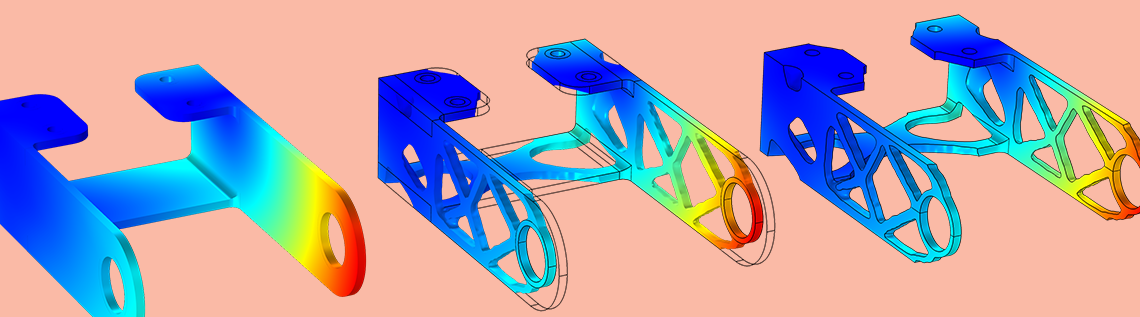# 通过密度方法进行拓扑优化

2019年 1月 4日

### 拓扑优化和密度方法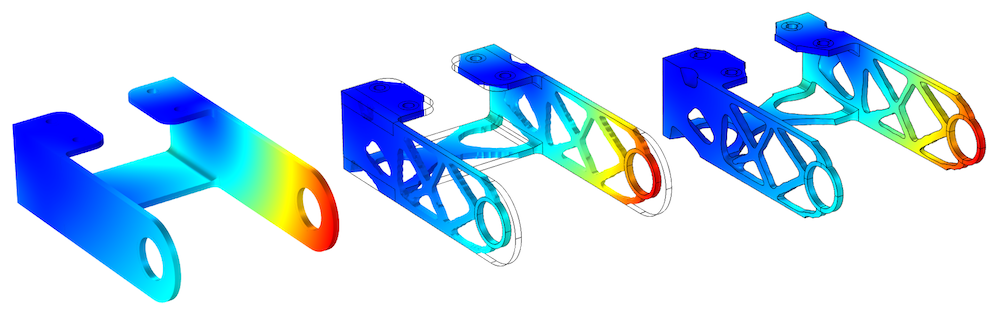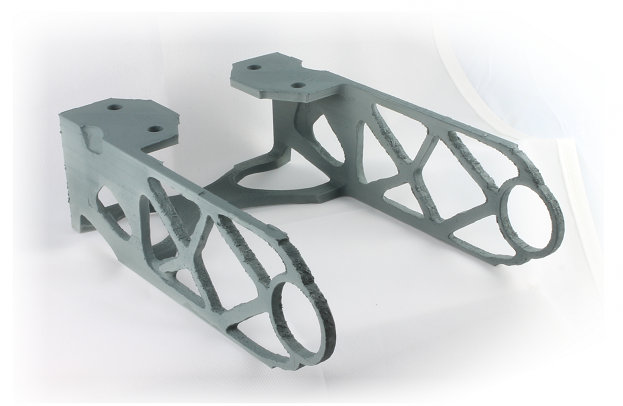\rho \frac{D\mathbf{v}}
{Dt}
= -\mathbf
{\nabla}p+\eta\nabla^2\mathbf{v}-\alpha(\theta_c)\mathbf{v}

### 密度模型功能简介

\theta_f = R_\mathrm{min}^2\mathbf{\nabla}
^2\theta_f + \theta_c.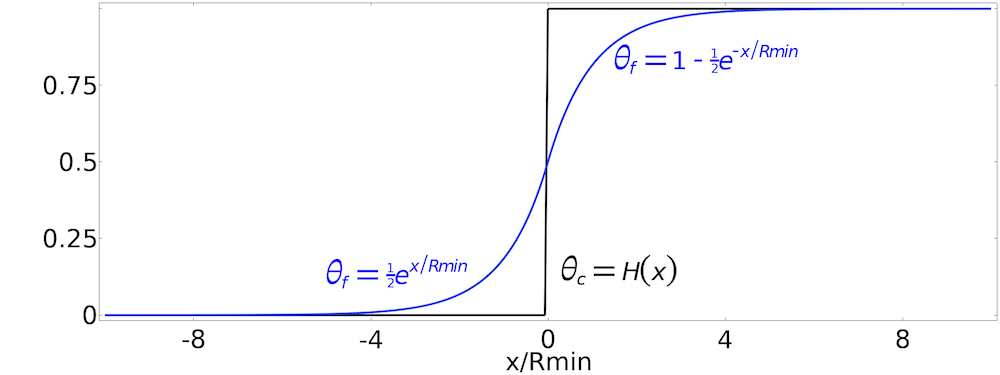\theta = \frac{\tanh(\beta(\theta_f-\theta_
{\beta}))+\tanh(\beta\theta_{beta}
)}{\tanh(\beta(1-\theta_
{\beta}))+\tanh(\beta\theta_{beta}
)}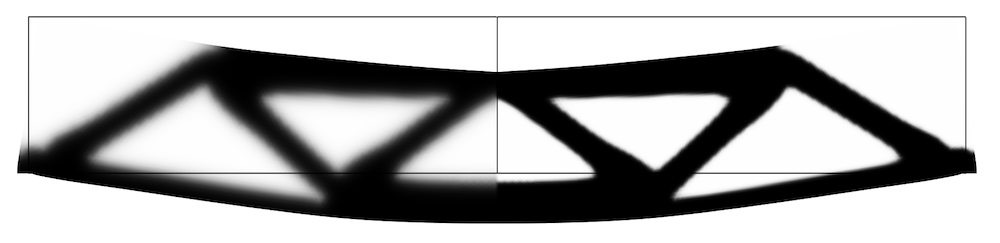\begin{align}
\theta_p %26= \theta_\mathrm{min}(1-\theta_\mathrm{min})\theta^{p_\textsc{simp}}\\
E_p %26= E\theta_p
\end{align}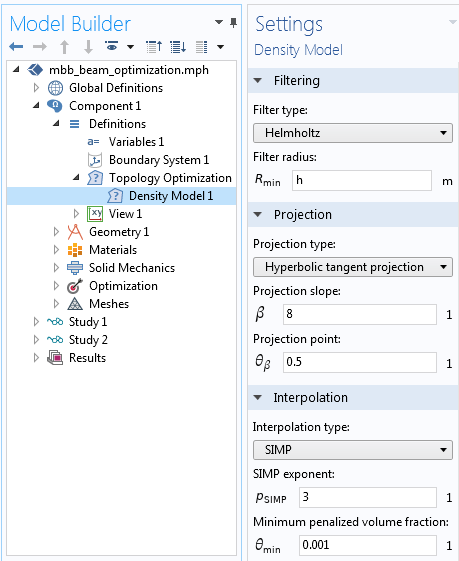“密度模型”功能位于组件 > 定义的拓扑优化下。网格边的长度被用作默认过滤器半径，并且效果很好，但是必须替换为固定值才能产生与网格无关的结果。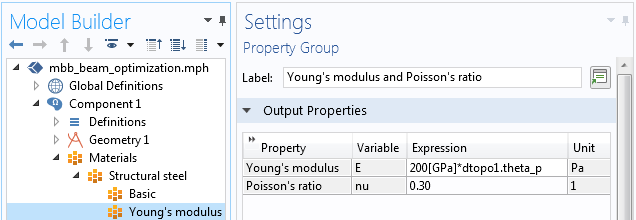\theta_c 边界控制材料体积因子 0\leq\theta_c\leq1
\theta_f 过滤的材料体积因子 \theta_f = R_\mathrm{min}^2\mathbf{\nabla}^2\theta_f + \theta_c
\theta 材料体积因子 \theta = \frac{\tanh(\beta(\theta_f-\theta_{beta}
))+\tanh(\beta\theta_

{\beta})}{\tanh(\beta(1-\theta_{beta}
))+\tanh(\beta\theta_

{\beta}
)}

\theta_p 罚材料体积因子 \theta_p = \theta_\mathrm{min}+(1-\theta_\mathrm{min}
)\theta^{p_\textsc{simp}}
或者 \theta_p = \frac{q_\mathrm{Darcy}(1-\theta)}{q_\mathrm{Darcy}
+\theta}

### 应用连续性以避免局部极小值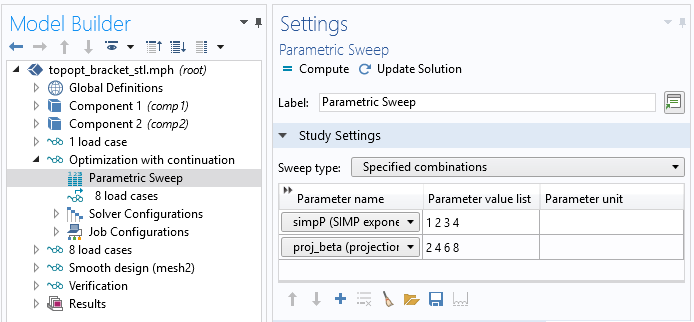### 拓扑优化中的目标和约束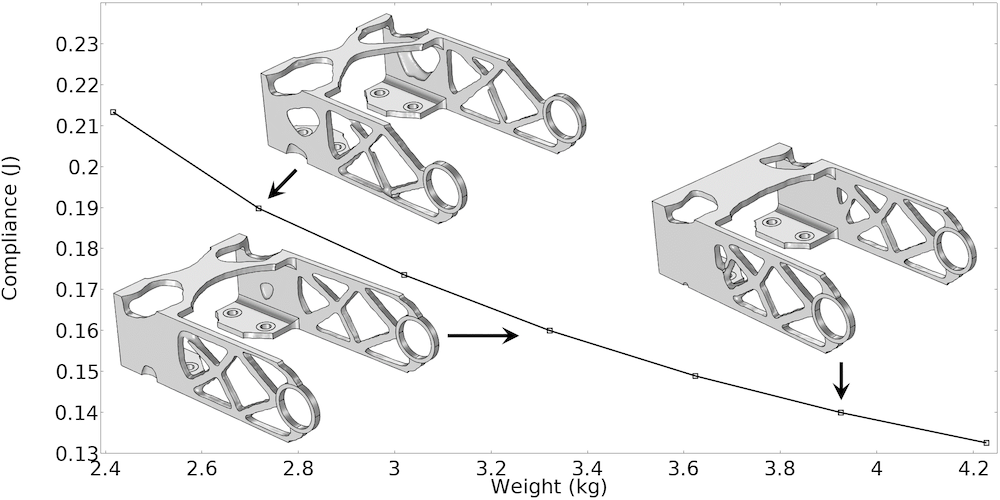### 导出和导入拓扑优化结果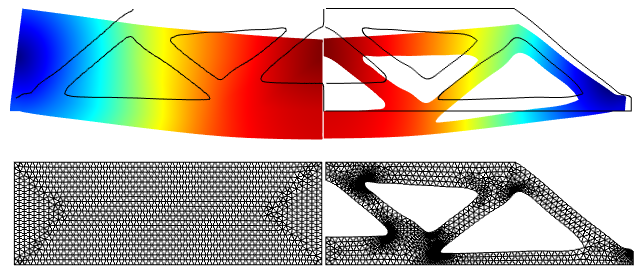### 后续操作

#### 更多资源

• 尝试对以下示例模型使用密度函数进行拓扑优化：
• 在 COMSOL 博客中了解有关拓扑优化的更多信息：
1. B.S. Lazarov and O. Sigmund, “Filters in topology optimization based on Helmholtz‐type differential equations,” International Journal for Numerical Methods in Engineering, vol. 86, no. 6, pp. 765–781, 2011.
2. F. Wang, B.S. Lazarov, and O. Sigmund, “On projection methods, convergence and robust formulations in topology optimization,” Structural and Multidisciplinary Optimization, vol. 43, pp. 767–784, 2011.
3. M.P. Bendsøe, “Optimal shape design as a material distribution problem,” Structural Optimization, vol. 1, pp. 193–202, 1989.

glTF 和 glTF 徽标是 Khronos Group Inc. 的商标。